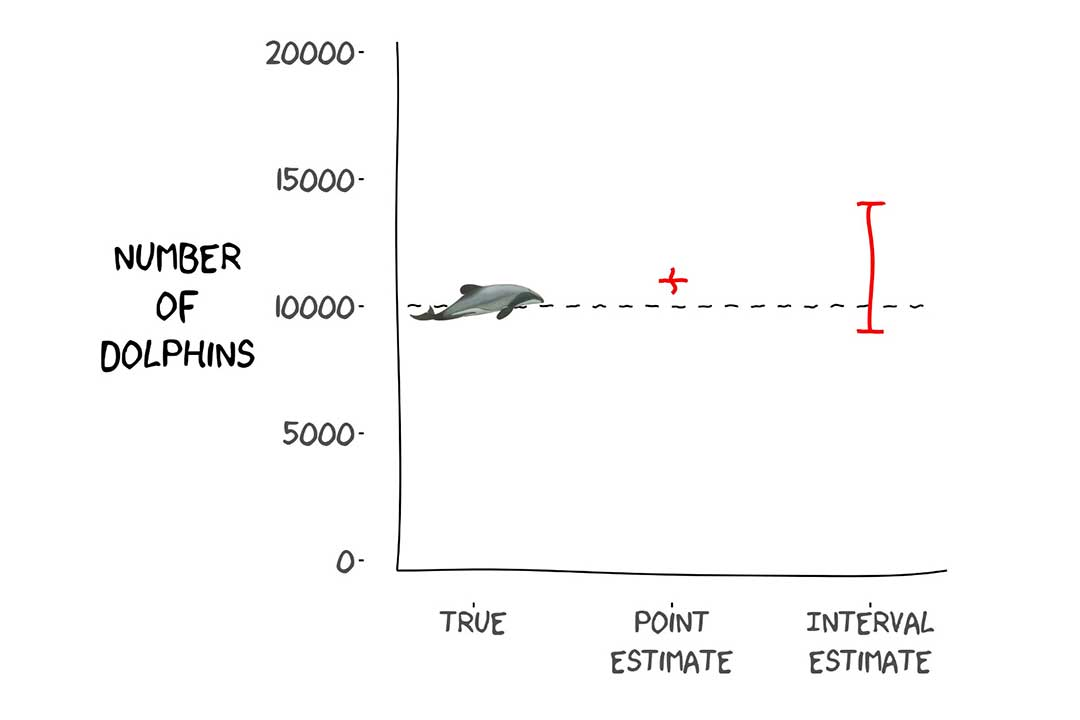## Confidence Intervals

In this month’s video tip Darryl explains how we should interpret confidence intervals.

As stated in the video, confidence intervals are a form of interval estimator, and the confidence level (“X% confidence”) is a property of the estimator (i.e., the estimation method), but that property shouldn’t be applied to our interpretation of an estimate that has been calculated for an actual set of data. Much like for a point estimator which may be unbiased (which is the property of the point estimator), but the estimate we obtain from an analysis isn’t expected to have exactly the right value.

The following are two common misinterpretations of confidence interval estimates.

“There is a 0.95 probability that a 95% confidence interval does contain the true value for the quantity of interest.”

This is incorrect because once the confidence interval estimate has been calculated, it either does or doesn’t contain the true value; there is no probability associated with that outcome, it’s either yes or no. It will only be reasonable to make a statement like this before we’ve collected the data and calculated the confidence interval.

“There is a 0.95 probability the true value will be within the 95% confidence interval.”

While this is an a priori statement (before data has been collected), it is not strictly correct because it implies the true value is variable and the interval is fixed, whereas the opposite is the case (at least according to a frequentist view of statistics).

Check out the video for how we should be doing it (skip to the 3:55 mark if you want to go straight to the punch line).